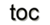Next: 2 Theoretical Concepts Up: 1 Introduction Previous: Motivation

# Organization

Part II is based on the gradual shift of several concepts into an abstract form for scientific computing. The starting point in Section 1.2 is given by a brief review of basic treatment of physical fields and in particular Maxwell's equations. The thereby given continuous formulations are then the basic motivation to introduce concepts and mechanism for the transition to the finite regime of computational methods. A basic topological toolkit (Section 1.3) is therefore introduced to enable structural properties of discrete spaces and is based on the work of Jänich  for common topological terms, Berti  for cell complex theory, and Zomorodian  for the computational topology (Section 1.4). Concepts of fiber bundles (Section 1.5) are adopted to enable a separation for the concepts given based on the work of Butler [20,21]. Algebraic topology  and differential forms [23,24,25] are established to analyze various discretization schemes [26,27,28,29,30], given in Section 2, to numerically deal with the given continuous problems. The discrete mapping is then given by concepts related to chains and cochains related to physical field theories and follows mainly the works of Tonti [31,32,24], Mattiussi [33,34,35,36], and Hyman . Furthermore, the formal handling of field theory and the application of algebraic topology to identify the underlying space describe the nature of different discretization methods.

Part III deals with the transformation of the presented theoretical concepts into generic and reusable software components. An important step, the C++ STL, is presented and analyzed as the beginning of a systematic development towards a reusable software component catalog . Various programming paradigms are presented in Section 4.2 and their related advantages and disadvantages [39,40,41,42,43,44,45] and performance issues  are illustrated. To enable efficient algorithm specification, concepts for domain-specific languages  are introduced in Section 4.3, whereas the focus of application design is shifted to the field of active libraries [47,48,49,50]. Section 4.4 reviews various works and assembles a set of requirements for generic software components. A topological generalization of data structures and a related common specification mechanism is then developed and introduced in Section 5. This part is heavily influenced by the works of Berti [18,51]. Utilizing the fiber bundle model a data model for application design is developed, based on concepts from scientific visualization  but with a special focus on application design. Finally, the remainder of Section 5 identifies necessary requirements for generic library-centric application design in the field of scientific computing.

Based on these theoretical concepts and practical concepts, Part IV introduces a set of generic components that operate on any data represented in the given models, represented by a generic scientific simulation environment, given in Section 7. A performance analysis is presented in Section 7.4 to highlight that multi-paradigm library-centric application design can easily compete with highly specialized applications. Concept-based programming and iterative refinement of algorithms is inspired by the work of Stepanov . Various applications present the applicability and feasibility of the presented approach of the generic scientific simulation environment, given in Section 8 and Section 9.Next: 2 Theoretical Concepts Up: 1 Introduction Previous: Motivation

R. Heinzl: Concepts for Scientific Computing